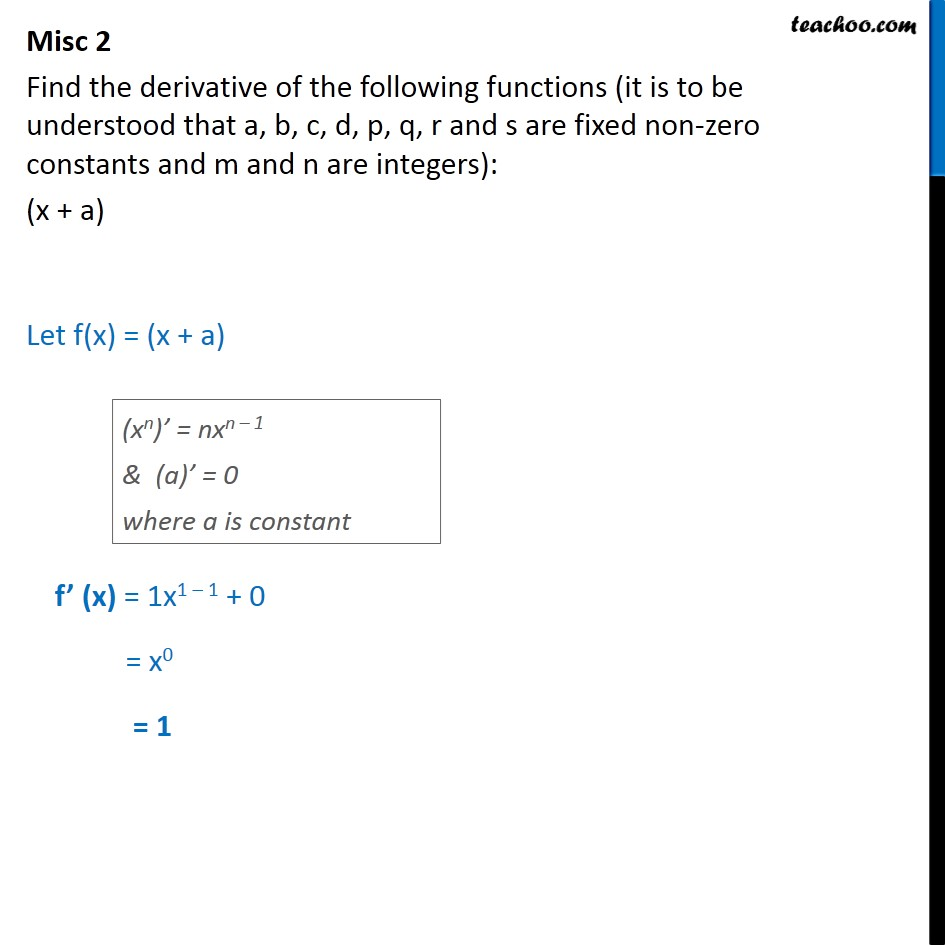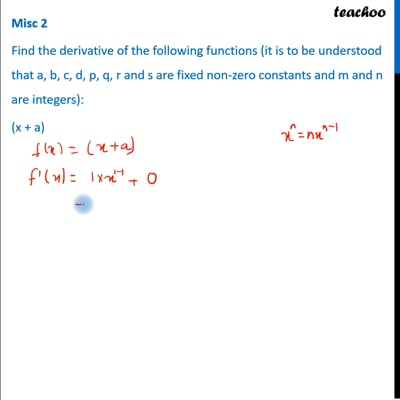Miscellaneous

Chapter 12 Class 11 Limits and Derivatives
Serial order wiseThis video is only available for Teachoo black users

Learn in your speed, with individual attention - Teachoo Maths 1-on-1 Class

### Transcript

Misc 2 Find the derivative of the following functions (it is to be understood that a, b, c, d, p, q, r and s are fixed non-zero constants and m and n are integers): (x + a) Let f(x) = (x + a) f’ (x) = 1x1 – 1 + 0 = x0 = 1